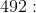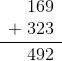Common Core: 2nd Grade Math : Add and Subtract Within 1000: CCSS.Math.Content.2.NBT.B.7

Example Questions

Example Question #1 : Add And Subtract Within 1000: Ccss.Math.Content.2.Nbt.B.7

Solve the following: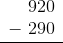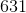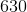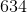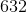Explanation:

When we subtract multi-digit numbers, we start with the digits in the ones place and move to the left.

Let's look at the numbers in the ones place: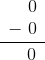Next, let's look at the numbers in the tens place: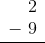When the top number is smaller than the bottom number, we have to borrow from the number to the left because we can't takeaway fromsinceis the smaller number. In this case, we are going to look to the. We only ever need to takeaway from the number to the left. For this problem, that will leave us with ato replace the. So far, your work should look something like this: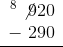Remember, we've borrowedfrom the hundreds place, so we can put ain front of the number in the tens place. So far, your work should look something like this: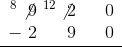Now, we can subtract the numbers in the tens place: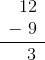Next, we can subtract the numbers in the hundreds place: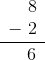Your final answer should be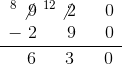Example Question #2 : Add And Subtract Within 1000: Ccss.Math.Content.2.Nbt.B.7

Solve the following: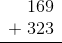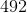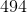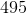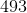Explanation:

When we add with multi-digit numbers, we start with the numbers in the ones place, and then move to the left to the tens place, followed by the hundreds place.

Add the numbers in the ones place: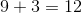Because this sum is greater than, we write thefrom the ones place and carry thefrom the tens place over to the left. Your work should look something like this: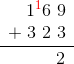Add the numbers in the tens place, including thethat was carried over: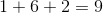Add the numbers in the hundreds place: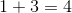Your final answer should be